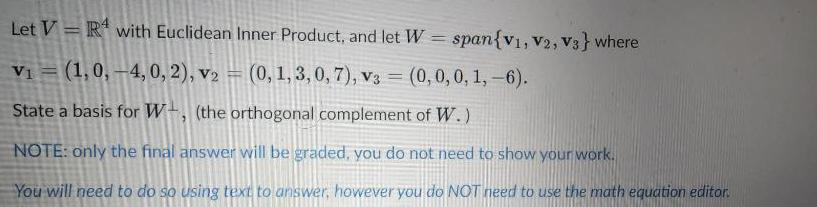Question:

# Let VR with Euclidean Inner Product, and let W = span{V1,

Last updated: 7/4/2022Let VR with Euclidean Inner Product, and let W = span{V1, V2, V3} where V₁ (1,0,-4, 0, 2), v2 = (0, 1, 3, 0, 7), v3 = (0, 0, 0, 1,-6). = State a basis for W, (the orthogonal complement of W.) NOTE: only the final answer will be graded, you do not need to show your work. You will need to do so using text to answer, however you do NOT need to use the math equation editor.